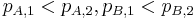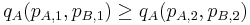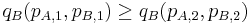# Law of joint demand for two goods

The law of joint demand for two goods is a variant for two goods of the law of demand.

## Statement

The law of joint demand for two goods says that if we have:$p_{A,1} < p_{A,2}, p_{B,1} < p_{B,2}$

then at least one of these must hold:$q_A(p_{A,1}, p_{B,1}) \ge q_A(p_{A,2}, p_{B,2})$$q_B(p_{A,1}, p_{B,1}) \ge q_B(p_{A,2}, p_{B,2})$

In other words, it cannot happen that the quantity demanded increases for both goods when both their increases.

When the goods are complementary goods, then in fact, both the above inequalities hold (i.e., both quantities demanded decrease or stay the same). However, when the goods are substitute goods, it is possible for the quantity demanded to increase for one of them when both prices increase. This is because the increase in price of one good pushes some of the demand for it to the other good.

The law of joint demand places a constraint on the nature of the demand surface.